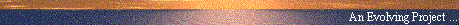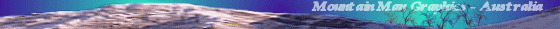# Averaging Cannot Yield Isotropy

#### Tom Roberts (sci.physics)

##### Web Publication by Mountain Man Graphics, Australia# Averaging Cannot Yield Isotropy

Date: Sun, 31 May 1998 14:20:33 -0500
From: Tom Roberts
Newsgroups: sci.physics.relativity
Subject: Averaging Cannot Yield Isotropy

```

Several people on this newsgroup have proposed theories to 'replace' SR, in which
the 1-way speed of light is not isotropic in every inertial frame, but in which
the 1-way speeds are correlated in such a way that by averaging the out and return
1-way speeds, the 2-way speed of light is isotropic in every frame. There are even
papers in the scientific literature proposing this sort of thing, most of which
claim that clock synchronization conventions other than Einstein's can give
different 1-way speed of light while still describing all experimental evidence.

These theories and proposals share a rather elementary error: they forget that
space is 3 dimensional! Without exception they deal only with motion along a
single axis.

This article will show that it is impossible to have an isotropic 2-way speed
of light without also having an isotropic 1-way speed of light (while retaining
ordinary notions of Euclidean space, vectors, etc.). We will also see a rather
amazing consequence of such claims which nobody else seems to have noticed:
if the 1-way speed were not isotropic but the 2-way speed is, then there is a
strict limit on the 1-way speed of light. This presumably imposes restrictions
on the reference systems available to material bodies....

Notation: I will use capital 'C' to mean some speed of light, and will subscript
it with lower-case letters to indicate which speed of light it represents. I will
assume there is a single absolute reference frame in which the speed of light
is isotropic, but that it need not be isotropic in frames moving wrt this one. In
_ALL_ cases, every reference frame or coordinate system I use will be inertial. I
have no idea how to single out this absolute frame; I merely postulate its
existence, and assume that any frame's motion can somehow be related to the
absolute frame (actually all that is needed is the direction of motion).

In the absolute frame:

Cabs	the (isotropic) speed of light		[not used]

In a frame moving wrt the absolute frame:

Cf	the forward 1-way speed of light (in the direction of motion)
Cb	the backward 1-way speed of light (opposite to the direction of motion)
Crt	the round-trip speed of light, for a given path
Cp	the perpendicular speed of light (perpendicular to the direction of motion)

Let us consider a given reference frame in uniform (inertial) motion wrt the
absolute frame. Let us erect coordinates such that the direction of motion is
along the +X axis. All measurements will be made in this frame.

Note carefully: the only reference to the postulated absolute frame
is the direction of motion between this absolute frame and the frame
used for calculations. There is no need to transform from one frame
to another. All calculations are done in this one frame.

First note that the cylindrical symmetry of the physical situation ensures that
Cp is independent of direction (as long as it remains perpendicular to the X axis).
This also implies that Cp is both the 1-way and the 2-way speed of light
perpendicular to the direction of motion. Thus, I can simply choose a plane at
arbitrary azimuth around the X axis to work in; let us designate this the X-Y
plane, with the Z axis perpendicular to this plane (Z is unused).

Now if we are to have an isotropic round-trip speed of light, then the round-trip
speed of light along the X axis must equal Cp. The time taken for light to travel
forward and backward along a path of length L along the X axis is:

delta-T = L/Cf + L/Cb

The average speed of light over this round-trip path is:

Crt = 2 L / delta-T
Crt = 2 Cf Cb / (Cf + Cb)			

So we must have Crt for this path equal to Cp. Putting this in and solving for Cb:

Cb = Cp Cf / (2 Cf - Cp)			

Thus we have a single free parameter: Cf. Cb is determined by the requirement 
that the round-trip speed of light be the same parallel to and perpendicular to
the direction of motion. Note that if Cf = Cp, then Cb = Cp and the 1-way speed
of light is the same parallel to and perpendicular to the direction of motion.

So far there is no problem -- one can set Cf to any appropriate value, and obtain
the same round-trip speed of light for perependicular paths as for paths parallel
to the direction of motion. But what are "appropriate" values for Cf? Looking at
, it is clear that Cf = Cp / 2 causes problems -- Cb must then be infinite!
That is unphysical, and smaller values cause Cb to be negative (again
unphysical!). For some reason, none of the advocates of this idea have ever
commented on this rather strange limit. Nor have they discussed its implications
for limitations on the speed of a frame wrt the absolute frame....

Okay. Now let's consider a round-trip path of length L at an arbitrary angle
t (theta) wrt the X-axis in the X-Y plane. Let Cout be the outgoing speed, and
Cret be the return speed. For t < PI/2:

Cout = sqrt(Cf^2 cos^2(t) + Cp^2 sin^2(t))		
Cret = sqrt(Cb^2 cos^2(t) + Cp^2 sin^2(t))		

And we see that for t > PI/2 the same equations hold (addition is commutative),
so that restriction is not needed.

AND THERE IS A PROBLEM -- Snell's Law cannot hold. That is, if a light
beam is reflected from a mirror, then its incident angle cannot equal
its reflected angle, unless the mirror is parallel to or perpendicular
to the X axis. Don't ask me how momentum can be conserved....
But let's ignore this. Don't ask me what magic there is to make it
work -- this is NOT my theory! Let's just ASSUME that we can reflect
light rays back on their original path.

The time for this round-trip path is:

delta-T = L/Cout + L/Cret

And the round-trip speed is:

Crt = 2 L / delta-T = 2 / (1/Cout + 1/Cret)
Crt = 2 Cout Cret / (Cout + Cret)				
{this is rather messy to write out in ASCII, so I won't}

To obtain an isotropic round-trip speed of light, we must have Crt = Cp,
independent of angle t. Equation  for Crt is not independent of t, unless
Cf = Cp. That is, one cannot obtain an isotropic round-trip speed of light,
unless the 1-way speed of light is also isotropic.

Please note: all calculations have been performed in the single
frame. All calculations simply measure round-trip velocity in
the usual way (distance travelled / time elapsed). Only a single
clock is used, and there is therefore no dependence upon any clock
synchronization method.

To investigate this further, I computed values of Crt for 17 values of t from 0
thru PI, normalizing to Cp. I selected the value of Cf to be Cp times 1 minus
the ratio of the CMBR speed to the speed of light:

Cp = 1;   Cf = 0.9988  (1 - 360,000/300,000,000)
t (Theta)		    Crt / Cp
0.00000000000000	1.00000000000000
0.19634954084936	1.00000007927131
0.39269908169872	1.00000027064919
0.58904862254809	1.00000046202717
0.78539816339745	1.00000054129871
0.98174770424681	1.00000046202760
1.17809724509617	1.00000027064965
1.37444678594553	1.00000007927148
1.57079632679490	1.00000000000000
1.76714586764426	1.00000007927148
1.96349540849362	1.00000027064965
2.15984494934298	1.00000046202760
2.35619449019234	1.00000054129871
2.55254403104171	1.00000046202717
2.74889357189107	1.00000027064919
2.94524311274043	1.00000007927131
3.14159265358979	1.00000000000000

We see that as expected, t=0, t=PI/2, and t=PI all give the same value, because
we computed Cb to make that happen. We also see that for other angles the 2-way
speed of light is not this same value. By playing with the spreadsheet, I can see
that the aniosotropy is proportional to ((Cp-Cf)/Cp)^2 to lowest order, and that
there are higher-order terms present (there is not strict symmetry around PI/2).
This is also rather obvious from examining the equations themselves. Note also
that Crt is never less than 1; this remains true for Cf > Cp, because the
equations are completely symmetric in Cf and Cb.

And there is an interesting conclusion:

THE MICHELSON-MORLEY EXPERIMENT WOULD NOT OBSERVE THIS ANISOTROPY!

That's because the experiment's two arms at 90 degrees apart would always
see identical round-trip velocities independent of angle, and the fringes
would not move. This is to 2-nd order; the MMX could in principle see the
higher-order corrections, but they are much smaller than the experimental
resolution. To use an MMX-like experiment to rule out an anisotropy like this,
one should use arms at 45 degrees or 135 degrees (so one arm is at a maximum
while the other is at a minimum).

Hey! Is this new? Has anybody seen any mention of such a modification
to the MMX? Or seen any such results? I haven't, and I have been
looking in this area for some time....

Before jumping to the conclusion that such a theory might be viable, please
remember that a theory must describe all of the experimental evidence.
Brillet-Hall clearly rules out any such anisotropy, down to MUCH smaller
anisotropies than the CMBR speed.

Brillet and Hall rotated a single-mode laser while measuring the
beat frequency between the rotating laser and a fixed laser. The
frequency and wavelength of a laser are related by the round-trip
speed of light along the laser axis. Since their single-mode laser
did not jump modes, an anisotropy in Crt would directly cause
a variation in the frequency of the laser beam. Their observed
variations were many orders of magnitude smaller than those in
the table above.

In fact, an anisotropy in Crt as large as that in the table above would
essentially prevent single-mode lasers from working. That variation is on the
order of 1 part per million. I estimate this implies that a single-mode laser
would have to jump modes whenever it was rotated by a few microradians. The
earth's rotation them implies that such a laser could stay in one mode only
for a few tens of milliseconds at most. They do not do this.

In summary, one cannot construct a self-consistent theory of light propagation
in which the round-trip speed of light is isotropic but the 1-way speed
is not. The 3-dimensional nature of space and the inherent interrelationships
among rotated vectors prevents it. There are numerous experiments showing that
the 2-way speed of light is indeed isotropic to a high degree (in inertial
frames, of course).

Tom Roberts	(tjroberts@lucent.com
May 1998
```Usenet Index | Theories of Aether | Mountain Man Graphics | E-Mail